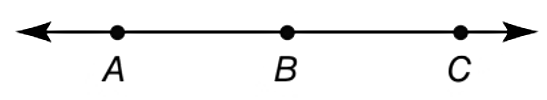Chapter P.1, Problem 8EElementary Geometry For College St...

7th Edition
Alexander + 2 others
ISBN: 9781337614085

Solutions

Chapter
SectionElementary Geometry For College St...

7th Edition
Alexander + 2 others
ISBN: 9781337614085
Textbook Problem

Let A , B , and C lie on a straight line as shown. Classify these claim as true or false.a) There is no point that lies between A and B .b) A B ¯ has endpoints A and B .To determine

a) To claim:

Whether true or false.

For the points A, B and C lie on a straight line shown, ‘There is no point that lies between A and B’.

Explanation

Calculation:

Given,

A, B and C are the points on a straight line.

Since, the line segment AB¯

To determine

b) To find:

Whether true or false.

For the points A, B and C lie on a straight line shown, ‘AB¯ has endpoints A and B’.

Still sussing out bartleby?

Check out a sample textbook solution.

See a sample solution

The Solution to Your Study Problems

Bartleby provides explanations to thousands of textbook problems written by our experts, many with advanced degrees!

Get Started

328

Applied Calculus for the Managerial, Life, and Social Sciences: A Brief Approach

Find the derivatives of the functions in Problems 1-10. 9.

Mathematical Applications for the Management, Life, and Social Sciences

Differentiate. y = sin cos

Single Variable Calculus: Early Transcendentals

For f(x) = 5 + g(x), f(x) = _____. a) 5g(x) b) 5 + g(x) c) 0 g(x) d) g(x)

Study Guide for Stewart's Single Variable Calculus: Early Transcendentals, 8th

For , f(x) = 0 2 3 f(3) does not exist

Study Guide for Stewart's Multivariable Calculus, 8th

Find x. logx32=5

College Algebra (MindTap Course List)